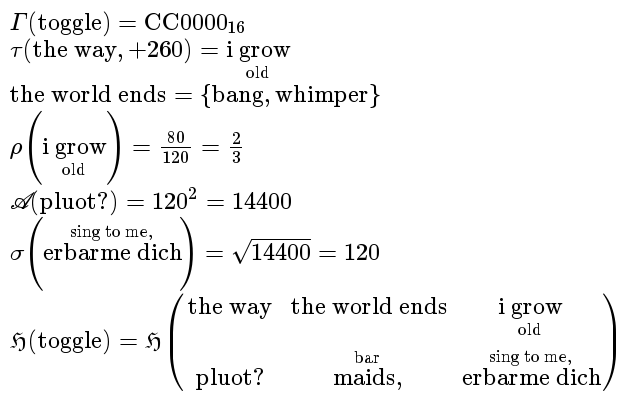# The MathML Acid1 test (description)

The MathML Acid1 test contains 7 mathematical expressions that should render more or less as follows:The basic requirements to pass the tests are:

1. In general, text is layout linearly from left to right with normal style (i.e. not italic), modulo the exception below.
2. All characters should be displayed properly, including greek, fraktur and script letters.
3. In test 1, "16" should be rendered as a subscript.
4. In test 2, 4 and 7, "old" should be rendered as an underscript.
5. In test 6 and 7 "sing to me," should be rendered as an overscript.
6. In test 7 "bar" should be rendered as an overscript.
7. In test 4, 80/120 and 2/3 should be rendered as fractions with numerator above denominator and horizontal fraction bar aligned with the equation axis.
8. In test 5, "2" should be rendered as a superscript.
9. In test 6, "square root of 44000" should be rendered as a radical symbol covering the "44000".
10. In test 7, elements "the way", "the world ends" etc should be layout as a table with 2 rows and 3 columns, vertically centered with respect to the equation axis.
11. The greek letters should be italic. Note that the fraktur h and script A are supposed to be invariant to style change, most fonts render the latter slanted.
12. The parentheses should stretch to become at least as tall as their content and should render symmetrically with respect to the equation axis.
13. The spacing around the operators, namely invisible function application U+2061, parenthesis, braces, plus and equal signs, should be "reasonable" in some sense (neither too small nor excessively large). These operators should also be correctly aligned with respect to the equation axis.